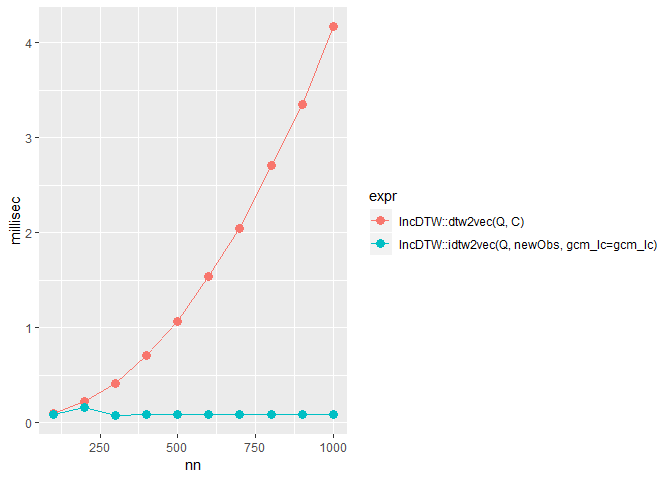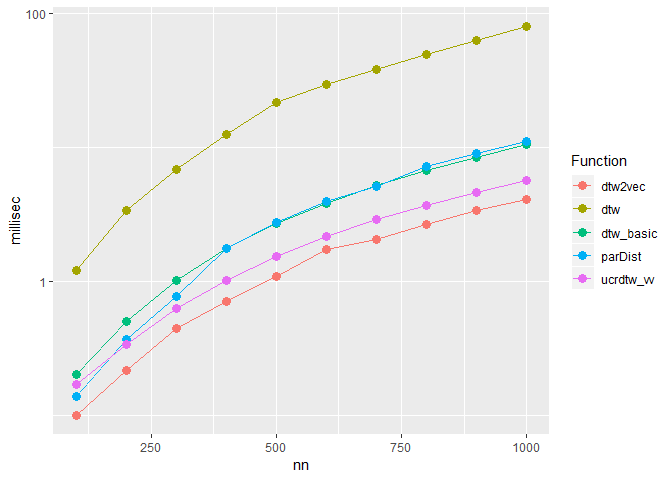# Calculation Time Comparison

We split the computation time comparisons by the 3 data analysis tasks: (1) incremental update for new observations, (2) single DTW computation for two time series, and (3) computing the matrix of pairwise DTW distances for a set of time series. To compare the calculation times we use the package microbenchmark. First we define the function cond_mic() to evaluate expressions conditionally to a time constraint. If the evaluation of the former iteration has hit a limit, the next iteration with larger input vectors is truncated. We use this function in all the following runtime experiments.

cond_mic <- function(expr, df, repetitions, threshold, nn){
if(df[df$expr == expr, "doit"]){ mic <- microbenchmark::microbenchmark(eval(parse(text = expr)), times = repetitions) mic <- data.frame(expr = expr, times = mic$time,
nn=nn, stringsAsFactors = FALSE)
if(median(mic$time) > threshold){ df[df$expr == expr, "doit"] <- FALSE
}
}else{
mic <- data.frame(expr = rep(expr, repetitions), times = NA,
nn=nn, stringsAsFactors = FALSE)
}
return(list(mic = mic, df = df))
}

## Incremental Update of DTW

The fastest way to compute the DTW distance measure is by recycling former calculated results. In the following we simulate the situation of continuously recording new observations and compare the run time for the incremental calculation with a traditional calculation from scratch.

First we define the expressions to be benchmarked and the function cond_mic() we use to test the expressions.

df <- data.frame(expr = c("IncDTW::dtw2vec(Q, C)",
"IncDTW::idtw2vec(Q, newObs, gcm_lc=gcm_lc)"),
doit = TRUE, stringsAsFactors = FALSE)

Next we run the for loop and evaluate the expressions for different vector lengths.

REPETITIONS <-  30
THRESHOLD <- Inf
mics <- list()
for(nn in seq(100, 1000, 100)){
set.seed(nn)
C <- cumsum(rnorm(nn))
Q <- cumsum(rnorm(nn))

# initial calculation
res0 <- IncDTW::idtw2vec(Q=Q, newObs = C, gcm_lc = NULL)

# incremental calculation for new observations
gcm_lc <- res0$gcm_lc_new newObs <- rnorm(1) for(i in 1:nrow(df)){ tmp <- cond_mic(df$expr[i], df, REPETITIONS, THRESHOLD, nn)
df <- tmp$df mics[[length(mics)+1]] <- tmp$mic
}
}

mics <- do.call(rbind, mics)

Finally we plot the results## Single Computations

To guarantee a fair comparison we set the step pattern equal ‘symmetric1’ (since rucrdtw::ucrdtw_vv() only supports ‘symmetric1’) and do not restrict the warping path. Again first we define the expressions to be benchmarked:

STEP_PATTERN <- "symmetric1"
dtw2vec0 <- function(C, Q){
IncDTW::dtw2vec(Q = Q, C = C, step_pattern = STEP_PATTERN) }

dtw0 <- function(C, Q){
dtw::dtw(C, Q, step.pattern = dtw::symmetric1, distance.only = TRUE)$distance } dtw_basic0 <- function(C, Q){ dtwclust::dtw_basic(C,Q, step.pattern = dtw::symmetric1)} ucrdtw_vv0 <- function(x,y){ rucrdtw::ucrdtw_vv(x,y, dtwwindow = 0.9999)$distance}

parDist0 <- function(mat){
parallelDist::parDist(x = mat, method = "dtw", step_pattern = STEP_PATTERN)}

df <- data.frame(expr = c("dtw2vec0(C, Q)",
"dtw0(C, Q)",
"ucrdtw_vv0(C,Q)",
"dtw_basic0(C,Q)",
"parDist0(mat)"),
doit = TRUE, stringsAsFactors = FALSE)

Now we run the computations:

REPETITIONS <-  30
THRESHOLD <- 60 * 10^9# 1 minute in nano seconds
mics <- list()
for(nn in seq(100, 1000, 100)){
set.seed(nn)
C <- cumsum(rnorm(nn))
Q <- cumsum(rnorm(nn))
res0 <- IncDTW::dtw(Q = Q, C = C, return_diffM = TRUE)
diffM <- res0$diffM cm <- abs(diffM) mat <- matrix(c(Q,C), nrow=2, byrow = TRUE) for(i in 1:nrow(df)){ RcppParallel::setThreadOptions(numThreads = 1) tmp <- cond_mic(df$expr[i], df, REPETITIONS, THRESHOLD, nn)
df <- tmp$df mics[[length(mics)+1]] <- tmp$mic
}
}

mics <- do.call(rbind, mics)

And plot the results:The only two methods using a vector-based implementations rucrdtw_vec() and IncDTW_vec() are significantly faster than the remaining functions.

## Compute a distance matrix

To cluster or classify a set of time series by their pairwise DTW distance measures we need a distance matrix. The function IncDTW::dtw_dismat() helps to get this matrix for a list of univariate or multivariate time series of possibly different lengths. The calculations can be performed single threaded ncores = 1 or multihreaded.

SP <- "symmetric1"
DM <- "norm2"
NORM <- FALSE
WS <- NULL
f0 <- function(lot){IncDTW::dtw_dismat(lot, ws = WS, step_pattern = SP, dist_method = DM, normalize = NORM,
ncores = 1, return_matrix = FALSE)$dismat} fcp <- function(lot){IncDTW::dtw_dismat(lot, ws = WS, step_pattern = SP, dist_method = DM, normalize = NORM, ncores = 3, return_matrix = FALSE, useRcppParallel = TRUE)$dismat}
frp <- function(lot){IncDTW::dtw_dismat(lot, ws = WS, step_pattern = SP, dist_method = DM, normalize = NORM,
ncores = 3, return_matrix = FALSE, useRcppParallel = FALSE)$dismat} fpp <- function(lot){parallelDist::parDist(x=lot, ws = WS, method = "dtw", step_pattern = dtw::symmetric1, normalize = "blabla",threads = 3)} df <- data.frame(expr = c("f0(lot)", "fcp(lot)", "frp(lot)", "fpp(lot_t)"), doit = TRUE, stringsAsFactors = FALSE) REPETITIONS <- 30 THRESHOLD <- 60 * 10^9# 1 minute in nano seconds NLOT <- 500 mics <- list() SEQ0 <- seq(100, 1000, 100) for(nn in SEQ0){ lot <- lapply(1:NLOT, function(i){ matrix(rnorm(nn*2, log(i),1), ncol = 2) }) lot_t <- lapply(lot, t) for(i in 1:nrow(df)){ tmp <- cond_mic(df$expr[i], df, REPETITIONS, THRESHOLD, nn)
df <- tmp$df mics[[length(mics)+1]] <- tmp$mic
}
}
mics <- do.call(rbind, mics)
dfp <- aggregate(times ~ nn+expr, data = mics, median)
dfp$sec <- dfp$times/10^9
dfp$Function <- factor(dfp$expr, levels = c( "fcp(lot)", "frp(lot)","f0(lot)", "fpp(lot_t)"))
levels(dfp\$Function) <- c("dtw_dismat_3Rcpp", "dtw_dismat_3R", "dtw_dismat_1", "parDist_3")
ggplot2::ggplot(dfp) + ggplot2::geom_line(ggplot2::aes(x = nn, y = sec,
group = Function, col=Function))+
ggplot2::geom_point(ggplot2::aes(x = nn, y = sec, col=Function), size=3) +
ggplot2::scale_y_log10()

dtw_dismat() is the standard function equivalent to dis_mat() without parallelization. dtw_dismat_3Rcpp() parallels via RcppParallel and dtw_dismat_3R() applies parallel, both with three cores (ncores=3). Except of additional run time costs for initiating the cores these two solutions almost need the same computation time. The function parDist_3() also uses three cores and applies parallelDist::parDist, and takes about 1.8 as long as dis_mat.